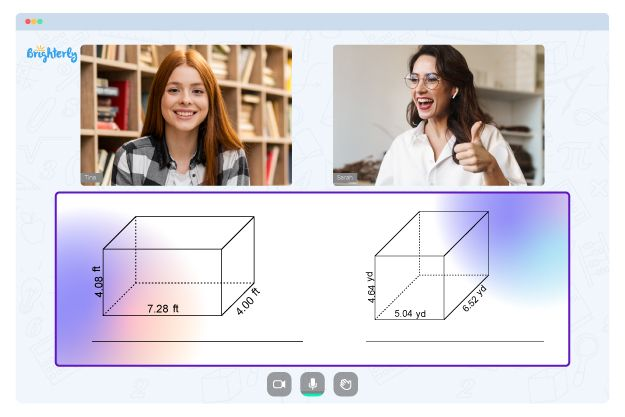# Parallel and Perpendicular Lines Worksheets

Identifying lines’ relative positions and angles to one another is part of learning geometry. The slopes of parallel and perpendicular lines worksheet helps students ace this part of their studies.

Kids can solve problems and exercises on parallel and perpendicular line worksheets, boosting their hands-on experience with geometry concepts. In this article, you will learn everything about parallel and perpendicular lines worksheet answers key geometry.

## Worksheets on Parallel Lines

The idea of parallel lines is fundamental to geometry and is necessary for comprehending numerous other mathematical concepts. They are defined as lines that will never cross and always have the exact distance between them. Practicing and reviewing parallel line worksheets are essential to get the full picture.

In the classroom, parallel line worksheets are an excellent resource for students and teachers. Students can practice and review math problems on parallel lines in an interactive way thanks to the variety of exercises and problems these tools offer. Teachers can evaluate their student’s comprehension of the material by using these worksheets as a form of assessment.

Math for Kids

Is Your Child Struggling With Math?
1:1 Online Math Tutoring## Worksheets on Perpendicular Lines

Perpendicular lines intersect at a right angle and are an essential concept in mathematics and geometry. In addition to being useful in real-world situations like engineering and construction, understanding perpendicular lines is critical for success in higher-level math classes. Different views are typically covered in the perpendicular line worksheets, including identifying, constructing, and resolving problems involving perpendicular lines.

Some examples of the problems in the worksheets are finding the slope of a line, determining the distance between two lines, or determining the area of a shape formed by perpendicular lines. Students can understand the role of lines in the real world and how to apply their knowledge to solve complex problems.

## Benefits of Parallel Perpendicular and Intersecting Lines Worksheet

Students’ geometry abilities can be enhanced using the parallel perpendicular and intersecting lines worksheet answer key. These math worksheets have practice exercises that get increasingly difficult to help students better understand the material. Students can differentiate between parallel and perpendicular lines by practicing with these worksheets.

Slopes of parallel and perpendicular lines worksheet answers help teach students to comprehend and practice working with lines. These worksheets prepare students for higher-level math classes by showing them how to recognize, construct, and solve problems involving perpendicular lines.Teachers and parents can use these worksheets for evaluation as well. Teachers can use the parallel and perpendicular lines worksheet answer key to assess their students’ comprehension of the concept and pinpoint areas in which they require additional assistance. Thanks to these resources, parents can tailor their kids’ educational activities to be more fluid and supportive.### Parallel and Perpendicular Lines Worksheets PDF

Parallel And Perpendicular Lines Worksheet Answers Key Geometry### Parallel and Perpendicular Lines Worksheets PDF

Parallel And Perpendicular Lines Worksheet### Parallel and Perpendicular Lines Worksheets PDF

Parallel Perpendicular And Intersecting Lines Worksheet### Parallel and Perpendicular Lines Worksheets PDF

Slopes Of Parallel And Perpendicular Lines Worksheet

Problems with Geometry?• Does your child struggle to grasp the concept of geometry?
• Try studying with an online tutor.

Is your child having difficulties with understanding geometry lessons? An online tutor could provide the necessary guidance.